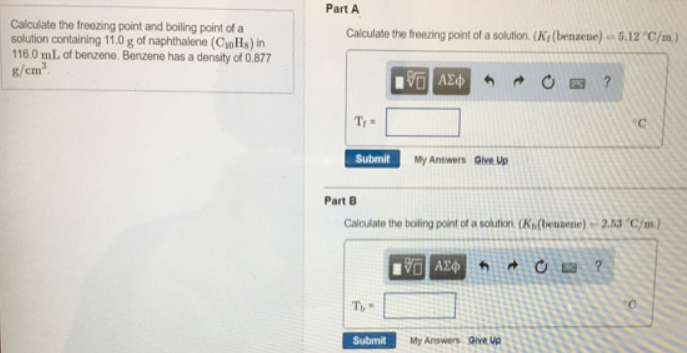# Problem: Calculate the freezing point and boiling point of a solution containing 11.0 g of naphthalene (C10H8) in 116.0 mL of benzene. Benzene has a density of 0.877 g/cm3. Part ACalculate the freezing port of a solution. (Kf (benzene) = 5.12  °C/m)Part BCalculate the boiling point of a solution (Kb (benzene) = 2.53  °C/m)

🤓 Based on our data, we think this question is relevant for Professor Jabbour's class at TOWSON.

###### FREE Expert Solution###### Problem Details

Calculate the freezing point and boiling point of a solution containing 11.0 g of naphthalene (C10H8) in 116.0 mL of benzene. Benzene has a density of 0.877 g/cm3

Part A

Calculate the freezing port of a solution. (Kf (benzene) = 5.12  °C/m)

Part B

Calculate the boiling point of a solution (Kb (benzene) = 2.53  °C/m)